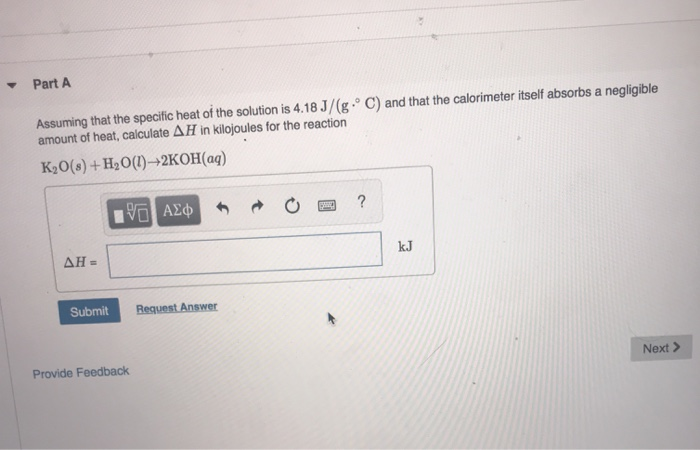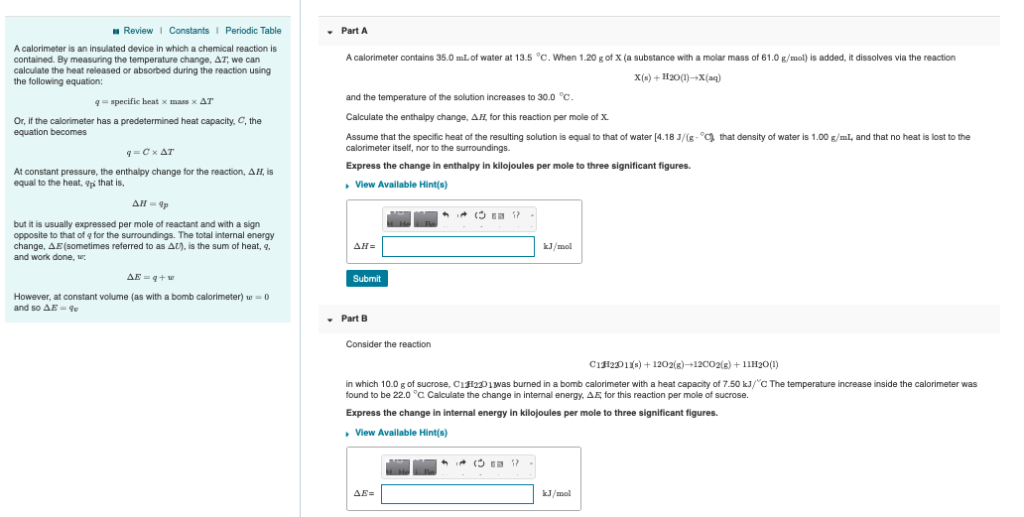# Calorimeter Chemistry Question

A calorimeter contains 950.0 g water that changes temperature by 11.3 °C when 1.00 g of diesel is burned. What is the heat of combustion of 1.00 g of diesel, in kilojoules?

The specific heat of water is 4.18 J/(g·°C). Remember that combustion is an exothermic chemical process. Please make sure that the sign of your answer indicates this. Round your answer to the tenths place.

-4.18 J/(g·°C) * 950.0g = 3,971 J/°C

-3,971 J/°C * 11.3 °C = 44,872.3 J

-44,872.3 J / 1,000 = -44.8 kJ

#### Earn Coin

Coins can be redeemed for fabulous gifts.

Similar Homework Help Questions
• ### When 0.187 g of benzene, C6H6, is burned in a bomb calorimeter, the temperature of both the water and the calorimeter r...

When 0.187 g of benzene, C6H6, is burned in a bomb calorimeter, the temperature of both the water and the calorimeter rises by 4.53 ∘C. Assuming that the bath contains 250.0 g of water and that the heat capacity for the calorimeter is 525 J/∘C , calculate the combustion energy (ΔE) for benzene in kilojoules per gram.

• ### When 0.187 g of toluene, C7H8, is burned in a bomb calorimeter, the temperature of both the water and the calorimeter r...

When 0.187 g of toluene, C7H8, is burned in a bomb calorimeter, the temperature of both the water and the calorimeter rises by 4.83 ∘C. Assuming that the bath contains 250.0 g of water and that the heat capacity for the calorimeter is 525 J/∘C, calculate the combustion energy (ΔE) for toluene in kilojoules per gram.

• ### Calorimeter question

A calorimeter contains 24.0 of water at15.0. When2.20 of (a substance with a molar mass of 69.0 ) is added, it dissolves via the reactionand the temperature of the solution increases to 26.0.Calculate the enthalpy change, , for thisreaction per mole of .Assume that the specific heat and density of the resulting solution are equal to those of water [4.18 and 1.00] and that no heat is lost to the calorimeter itself, norto the surroundings.Express the change in enthalpy in...

• ### Assuming that the specific heat of the solution is 4.18 J/(g⋅∘C) and that the calorimeter itself...Assuming that the specific heat of the solution is 4.18 J/(g⋅∘C) and that the calorimeter itself absorbs a negligible amount of heat, calculate ΔH in kilojoules for the reaction. Part A Assuming that the specific heat of the solution is 4.18 J/(g . C) and that the calorimeter itself absorbs a negligible amount of heat, calculate AH in kilojoules for the reaction K2O(8)+H20()-2KOH(aq) ανα ΑΣφ ? ΔΗ - kJ Request Answer Submit Provide Feedback Next> Constants Periodic Tab When 1.045...

• ### Part A: A calorimeter contains 26.0 mL of water at 13.0 ∘C . When 2.10 g...

Part A: A calorimeter contains 26.0 mL of water at 13.0 ∘C . When 2.10 g of X (a substance with a molar mass of 49.0 g/mol ) is added, it dissolves via the reaction X(s)+H2O(l)→X(aq) and the temperature of the solution increases to 25.0 ∘C . Calculate the enthalpy change, ΔH, for this reaction per mole of X. Assume that the specific heat of the resulting solution is equal to that of water [4.18 J/(g⋅∘C)], that density of water...

• ### ReviewI Constants1 Periodic Table Part A A calorimeter is an insulated device in which a chemical reaction is contained By measuring the temperature change. ΔΤ, we can calculate the heat released or...ReviewI Constants1 Periodic Table Part A A calorimeter is an insulated device in which a chemical reaction is contained By measuring the temperature change. ΔΤ, we can calculate the heat released or absorbed during the reaction using the following equation: A calorimeter contains 35.0 mL of water at 13.5 °C . when 1.20 g of X (a substance with a molar mass of 61.0 g/mol) is added, it dissolves via the reaction X+20)-X(ag) and the temperature of the solution increases...

• ### A calorimeter contains 27.0 mL of water at 14.0 ∘C . When 2.00 g of X...

A calorimeter contains 27.0 mL of water at 14.0 ∘C . When 2.00 g of X (a substance with a molar mass of 77.0 g/mol ) is added, it dissolves via the reaction X(s)+H2O(l)→X(aq) and the temperature of the solution increases to 26.5 ∘C . Calculate the enthalpy change, ΔH, for this reaction per mole of X. Assume that the specific heat of the resulting solution is equal to that of water [4.18 J/(g⋅∘C)], that density of water is 1.00...

• ### A calorimeter contains 27.0 mL of water at 14.0 ∘C . When 2.00 g of X...

A calorimeter contains 27.0 mL of water at 14.0 ∘C . When 2.00 g of X (a substance with a molar mass of 77.0 g/mol ) is added, it dissolves via the reaction X(s)+H2O(l)→X(aq) and the temperature of the solution increases to 26.5 ∘C . Calculate the enthalpy change, ΔH, for this reaction per mole of X. Assume that the specific heat of the resulting solution is equal to that of water [4.18 J/(g⋅∘C)], that density of water is 1.00...

• ### A calorimeter contains 35.0 mL of water at 12.0 ∘C . When 2.30 g of X...

A calorimeter contains 35.0 mL of water at 12.0 ∘C . When 2.30 g of X (a substance with a molar mass of 70.0 g/mol ) is added, it dissolves via the reaction X(s)+H2O(l)→X(aq) and the temperature of the solution increases to 26.5 ∘C . Calculate the enthalpy change, ΔH, for this reaction per mole of X. Assume that the specific heat of the resulting solution is equal to that of water [4.18 J/(g⋅∘C)], that density of water is 1.00...

• ### A calorimeter contains 19.0 mL of water at 11.5 ∘C . When 2.50 g of X...

A calorimeter contains 19.0 mL of water at 11.5 ∘C . When 2.50 g of X (a substance with a molar mass of 63.0 g/mol ) is added, it dissolves via the reaction X(s)+H2O(l)→X(aq) and the temperature of the solution increases to 30.0 ∘C . Calculate the enthalpy change, ΔH, for this reaction per mole of X. Assume that the specific heat of the resulting solution is equal to that of water [4.18 J/(g⋅∘C)], that density of water is 1.00...